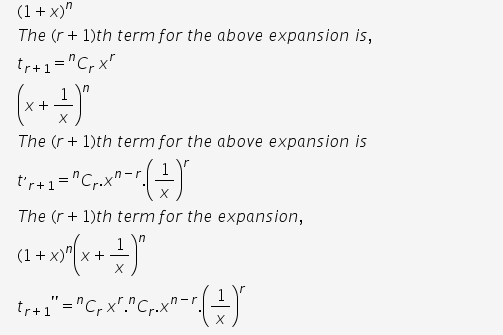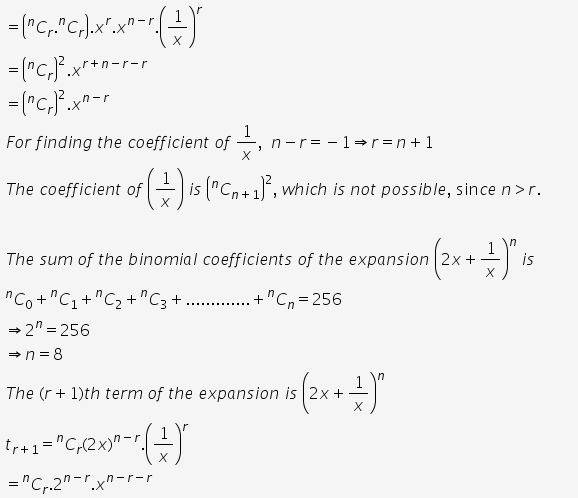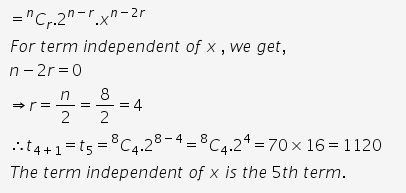# Find the coefficient of 1/x in the expansion of (1+x)^n(1/x+x)^n

Find the coefficient of 1/x in the expansion of (1+x)n(1/x+x)n
If the sum of binomial coefficients of the expansion (2x+1/x)n is equal to 256, then find the term independent of x